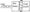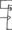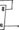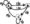# Logic design July 2008

Note : Answer any FIVE questions, choosing at least two from each part.

PART-A

1 a. Simplify the following expression using Karnaugh Map. Implement the simplified circuit using the gates as indicated.

i)   f (ABCD) = £m(2,3,4,5,l 3,15)+ 2ct(8,9,l 0,11) use only NAND gates

ii)   f(ABCD) = 7i(2,3,4,6,7,10,ll,12) use only NOR gates to implement these circuits.

b. Fig shows a BCD counter that produces a 4 -bit output representing the BCD code for the number of pulses that have been applied to the counter input. For example, after four pulses have occurred, the counter outputs are (ABCD) = (0100)2 = (04)io. The counter resets to 0000 011 the tenth pulse and starts counting over again. Design the logic circuit that produces a HIGH output. Whenever the count is 2, 3 or 9. Use K – mapping and take advantages of “don’t care” conditions. Implement the logic circuit using NAND gates.Fig.  Q  1(b)

2 a. Simplify the logic function given below, using Quine-McCluskey minimization technique. Y(ABCD)= Σ m{0,l,3,7,8,9,l 1,15). Realize the simplified expression using universal gates.

b. Simplify the logic function given below using variable – entered mapping (VEM) “‘Technique. Y(ABCD)= Sm(l,3J4,5,8,9,10,15) + Sd(2,7,ll,12,13).

3 a. Realize the following Boolean function f (ABCD) = S(0,l,3,5,7)

Using- i) 8 : 1 MUX(74151) ii) 4: 1 MIX(74153).

b. Design a combinational logic circuit that will convert a straight BCD digit to an Excess – 3 BCD digits,

i) Construct the truth table

ii) Simplify each output function using Karnaugh Map and write the reduced equations,

iii) raw the resulting logic diagram.

4 a. Design a 4 – bit BCD adder circuit.using 7483 IC chip, with self collecting circuit, i.e., a

provision has to be made in the circuit, in case if the sum of the BCD number exceeds 9.

b. Design a combinational circuit that accepts two unsigned 2 – bit binary number and provides 3 outputs. Inputs: word A = AjAo, word B = B1B0.

Output : A = B, A>B, A<B.

PART -B

5 a.Derive the characteristics equations of the following flip flops.

i) SR flip flops                    ii) JK flip flop.

b. Explain clearly the operation of an asynchronous inputs in a flip flops with suitable example.

c. An edge triggered ‘D’ flip flop is connected as shown in the Fig. Q 5(b). Assume that Q = 0 initially and sketch the wave form and determine its frequency of the signal at ‘Q’ output.Fig. Q 5(C)

6 a. With the help of a suitable example, explain the following operations in a shift register, i) SISO    ii) PISO   iii) Twisted ring counter.

b. Design a ripple counter to count the following sequence, 1111, 1110, 1101, 1100, 1011, 1111, 1110, 1101, 1100, 1011, etc. Suggest a suitable circuit using 7490 and other gates to obtain the desired result.

7 a. With a suitable example, explain the Mealy and Moore Model of a sequential circuit.

b. Construct the state table for the following state diagram.Fig. Q 7(c)

8 a. Design a clocked sequential circuit that operates according to the state diagram shown. Implement the circuit using D – flip flop.b. Design a counter using JK – flip flops whose counting sequence is 000, 001, 100, 110, 111,101, 000 etc. by obtaining its minimal sum equations.